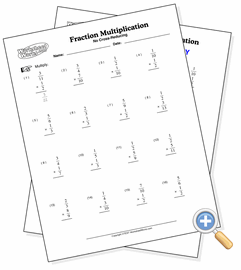# Without Reduction

## Fraction MultiplicationDevelop fraction multiplication skills not requiring reduction

These problems are the first that students new to fraction multiplication will need to learn. The result of the multiplication operation will not reduce so the only task here is to multiply both the numerators and denominators to have the result.